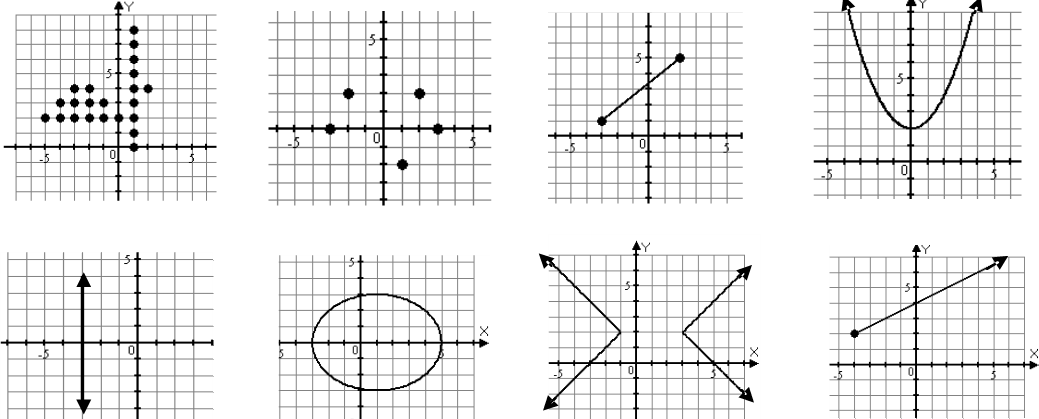# Identifying functions#### All in One Place

Everything you need for better grades in university, high school and elementary.#### Learn with Ease

Made in Canada with help for all provincial curriculums, so you can study in confidence.#### Instant and Unlimited Help

0/2
##### Intros
###### Lessons
1. What is a function?
2. How to do the Vertical Line Test?
1. Equations VS. Functions
0/7
##### Examples
###### Lessons
1. Are the following sets of ordered pairs functions?
1. (4,3), (2,6), (-3,4), (-2,5)
2. (4,5), (4,-3), (2,6), (3,2)
3. (3,6), (2,6), (5,3), (1,2)
2. Which of the following is a function?1. Using the table of values to answer the questions below:
1. X value -5 -3 -1 1 3
Y value 3 1 -1 -2 -3
Express the relations using ordered pairs, mapping notations and determine is it a function
2. X value -5 -3 -1 1 3
Y value 2 2 -1 -2 -3
Express the relations using ordered pairs, mapping notations and determine is it a function
3. X value -5 -5 -1 1 3
Y value 2 3 2 3 -3
Express the relations using ordered pairs, mapping notations and determine is it a function
###### Topic Notes
Bring on more grid papers! In this chapter, we're going to study functions. Functions are notations that tell us what the value of y is for every value of x. By carrying out the vertical line test, we are able to find out the relationships of ordered pairs.
Relations: Sets of ordered pairs ( x , y )

Function: For every value of x, there is a value of y. It will need to pass the vertical line test.

One-To-One Function: For every one value of x, there is only one value of y, and vice versa. It will need to pass both vertical and horizontal line test.

Vertical line Test: A vertical line that intersects the graph of the equation only once when moves from across on the x-axis.